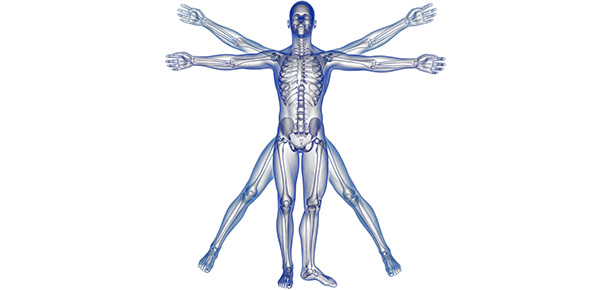# Physiology MCQ Quiz

211 Questions | Total Attempts: 56Settings.

Related Topics
• 1.
Compound action potentials can be graded responses.
• A.

True

• B.

False

• 2.
The sciatic nerve contains axons of both afferent and efferent neurones.
• A.

True

• B.

False

• 3.
The absolute refractory period of an action potential is the period after an initial stimulus when it is impossible to evoke another action potential with a second stimulus.
• A.

True

• B.

False

• 4.
The stimulus voltage required to give the maximal CAP amplitude represents the firing threshold of the most excitable axons in the nerve.
• A.

True

• B.

False

• 5.
The refractory periods of individual axons are important as they dictate the maximal action potential firing rate of a neuron.
• A.

True

• B.

False

• 6.
Action potential amplitude is proportional to the strength of the stimulus.
• A.

True

• B.

False

• 7.
Compound action potentials represent the algebraic sum of several individual action potentials being evoked at approximately the same time in a compound nerve.
• A.

True

• B.

False

• 8.
Upon reaching threshold, action potentials in single nerve fibres can be graded responses.
• A.

True

• B.

False

• 9.
The excitability threshold of an axon is proportional to its length.
• A.

True

• B.

False

• 10.
Intracellular electrical recording is often used as a tool for diagnosing problems with nerve conduction such as carpal tunnel syndrome.
• A.

True

• B.

False

• 11.
When applying an artificial electrical stimulus action potentials are initiated at the positive stimulating electrode.
• A.

True

• B.

False

• 12.
The time taken for an evoked compound action potential to reach a recording electrode is known as the CAP threshold.
• A.

True

• B.

False

• 13.
The sciatic nerve is the shortest mixed nerve in the frog.
• A.

True

• B.

False

• 14.
Within any given type of nerve fibre (myelinated or unmyelinated), conduction velocity is faster in large diameter fibres than in small diameter fibres.
• A.

True

• B.

False

• 15.
Conduction velocity in an axon is proportional to the strength of the stimulus.
• A.

True

• B.

False

• 16.
When recording a compound action potential from a nerve the threshold stimulus voltage represents the voltage at which the most excitable axons reach firing threshold.
• A.

True

• B.

False

• 17.
While recording a compound action potential using bipolar recording electrodes, the waveform of an evoked compound action potential can be converted from a biphasic to monophasic shape by tightly ligating the stimulated nerve after the most distal recording electrode.
• A.

True

• B.

False

• 18.
Compound action potentials are naturally occurring phenomena.
• A.

True

• B.

False

• 19.
In vivo, action potentials usually propagate bidirectionally.
• A.

True

• B.

False

• 20.
The relative refractory period roughly coincides with the period of action potential after hyperpolarisation.
• A.

True

• B.

False

• 21.
Intracellular fluid is sub extracellular fluid.
• A.

True

• B.

False

• 22.
Recruitment of motor units decreases the strength of contraction of skeletal muscle.
• A.

True

• B.

False

• 23.
The neuromuscular junction (NMJ) is a synapse.
• A.

True

• B.

False

• 24.
The action potential in skeletal muscle travels through the muscle via T-tubules
• A.

True

• B.

False

• 25.
The arrival of an action potential at the neuromuscular junction (NMJ) causes the release of noradrenaline.
• A.

True

• B.

False